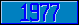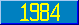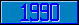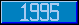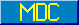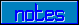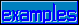## charset M

### Table A.1 - Character Set M

The charset M is defined using the following table. The values in columns headed Decimal and Character are taken from ASCII (X3.4–1990). The column headed patcode defines which characters match the patcodes A, C, E, L, N, P, and U. The characters which are defined as ident are those in the table with a patcode of A. Note that patcode E matches any character, not just those listed in this charset.

DecimalCharacterpatcode
0 NUL C,E
1 SOH C,E
2 STX C,E
3 ETX C,E
4 EOT C,E
5 ENQ C,E
6 ACK C,E
7 BELL C,E
8 BS C,E
9 HT C,E
10 LF C,E
11 VT C,E
12 FF C,E
13 CR C,E
14 SO C,E
15 SI C,E
16 DLE C,E
17 DC1 C,E
18 DC2 C,E
19 DC3 C,E
20 DC4 C,E
21 NAK C,E
22 SYN C,E
23 ETB C,E
24 CAN C,E
25 EM C,E
26 SUB C,E
27 ESC C,E
28 FS C,E
29 GS C,E
30 RS C,E
31 US C,E
32 SP (space) P,E
33 ! P,E
34 " P,E
35 # P,E
36 \$ P,E
37 % P,E
38 & P,E
39 ' (apostrophe) P,E
40 ( P,E
41 ) P,E
42 * P,E
43 + P,E
44 , (comma) P,E
44 - (hyphen) P,E
46 . P,E
47 / P,E
48 0 N,E
49 1 N,E
50 2 N,E
51 3 N,E
52 4 N,E
53 5 N,E
54 6 N,E
55 7 N,E
56 8 N,E
57 9 N,E
58 : P,E
59 ; P,E
60 < P,E
61 = P,E
62 > P,E
63 ? P,E
64 @ P,E
65 A A,U,E
66 B A,U,E
67 C A,U,E
68 D A,U,E
69 E A,U,E
70 F A,U,E
71 G A,U,E
72 H A,U,E
73 I A,U,E
74 J A,U,E
75 K A,U,E
76 L A,U,E
77 M A,U,E
78 N A,U,E
79 O A,U,E
80 P A,U,E
81 Q A,U,E
82 R A,U,E
83 S A,U,E
84 T A,U,E
85 U A,U,E
86 V A,U,E
87 W A,U,E
88 X A,U,E
89 Y A,U,E
90 Z A,U,E
91 [ P,E
92 \ P,E
93 ] P,E
94 ^ P,E
95 _ (underscore) P,E
96 ` P,E
97 a A,L,E
98 b A,L,E
99 c A,L,E
100 d A,L,E
101 e A,L,E
102 f A,L,E
103 g A,L,E
104 h A,L,E
105 i A,L,E
106 j A,L,E
107 k A,L,E
108 l A,L,E
109 m A,L,E
110 n A,L,E
111 o A,L,E
112 p A,L,E
113 q A,L,E
114 r A,L,E
115 s A,L,E
116 t A,L,E
117 u A,L,E
118 v A,L,E
119 w A,L,E
120 x A,L,E
121 y A,L,E
122 z A,L,E
123 { P,E
124 | P,E
125 } P,E
126 ~ P,E
127 DEL C,E

The exact collation value of a string is not defined here. However the collating value for any implementation must satisfy the following rules when used for comparing two strings:

Let s be any non-empty string, let m and n be strings satisfying the definition of numeric data values (see I.7.1.4.3), and u and v be non-empty strings which do not satisfy this definition. Then the collating ordering function CO is:

1. CO("",s) = s.
2. CO(m,n) = n if n > m; otherwise, CO(m,n) = m
3. CO(m,u) = u
4. CO(u,v) = v if v ] u where the ordering characters is determined by the relative positions in the table above; otherwise CO(u,v) = u.

In words, all strings follow the empty string, canonic numeric strings collate in numeric order, numerics precede nonnumeric strings, and nonnumeric strings are ordered by the ASCII value of their characters.